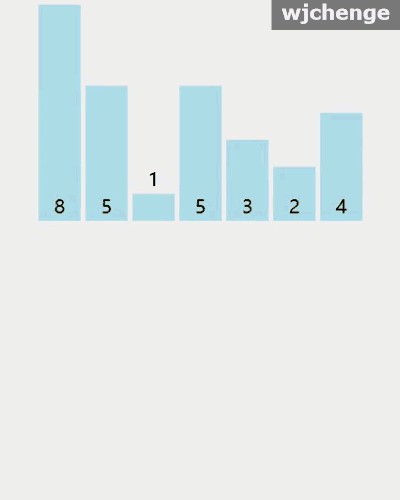# 插入排序wjchenge﻿﻿

Java代码实现如下：

public static void insertionSort(int[] a) {        if (a == null || a.length == 0) return;        for (int i = 1; i < a.length; ++i) {            int j = i;            int value = a[i];            //待排序的元素与已排好序区间的元素一一比较找到合适的位置            while (j > 0 && value < a[j-1]) {                //已排序元素依次往后移                a[j] = a[--j];            }            //将待排序元素值放入到合适位置            a[j] = value;        }    }

﻿

﻿## 评论